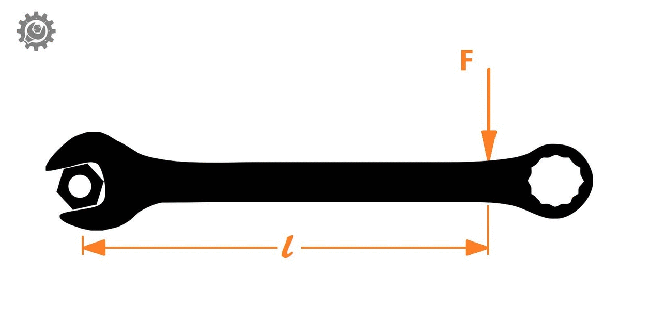• SECTION 01

(a)(i) Define the turning effect of force and give its SI Unit

The turning effect or moment of a force is a measure of its tendency to cause a body to rotate about a specific point or axis. Its obtained as the product of force and perpendicular distance from its line of action. The SI unit for moment of force is Newton Meter (Nm).Moment = Force x Distance (l) = (F)(l)

(ii) How can the moment of force be increased considerably in practical life? Give two examples.

There are two ways of increasing moment of force either by increasing the applied force or the perpendicular distance from center of moment. For most of practical cases increasing distance from the center of the rotation is the most preferred option, the following are examples of increased moment based on increase of perpendicular distance. Example 1: Longer spanners are more efficient for unscrew nuts than shorter spanners Example 2: Pushing a door further from its hinges is relatively easy compare to pushing it near its hinges.

• SECTION 02

(b)(i) List two factors that affects the stability of a body

• The location of center gravity of relative to the base

… the higher it is from its base, the less unstable

• The size(area) of the base compared to the body

… the larger it compared to the body, the more stable

(ii) Briefly explain why the handle of the door is near its outside edge?

Door hinges always located at near ends of the door in order to increase the turning effect of force on the door during opening and closing of door.

• SECTION 03

(c)(i) What is meant by a balanced beam?

A balanced beam is a solid supported bar such that the net momentum and resultant force acts upon it are both zero.

(ii) A uniform rod AB of mass 6.0g is balanced horizontally about a knife edge at a distance of 3cm form end A where a mass of 8.0g is hanging. Find the length of the rod.

Sum of clockwise moments = Sum of anti-clockwise moments

g = acceleration due to gravity

(0.006g N x X) = (0.008g N x 0.03m)

X = (0.008g N x 0.03m)/ 0.006g N = 0.04 m = 4 cm

But L = 2(X + 3)cm = 2(4cm + 3cm) = 14 cm

Length of rod is 14 cm.